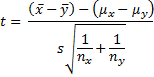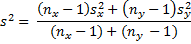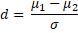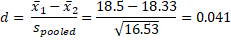# Two Sample t Test: equal variances

We now consider an experimental design where we want to determine whether there is a difference between two groups within the population. For example, let’s suppose we want to test whether there is any difference between the effectiveness of a new drug for treating cancer. One approach is to create a random sample of 40 people, half of whom take the drug and half take a placebo. For this approach to give valid results it is important that people be assigned to each group at random. Such samples are independent.

When the population variances are known, hypothesis testing can be done using a normal distribution, as described in Comparing Two Means when Variances are Known. But population variances are not usually known. The approach we use instead is to pool sample variances and use the t distribution.

We consider three cases where the t distribution is used:

• Equal variances
• Unequal variances
• Paired samples

We deal with the first of these cases in this section.

Theorem 1: Let  and ȳ be the sample means of two sets of data of size nx and ny respectively. If x and y are normal, or nx and ny are sufficiently large for the Central Limit Theorem to hold, and x and y have the same variance, then the random variablehas distribution T(nx + ny – 2) whereObservations, as defined above, can be viewed as a way to pool sx and sy, and so s2is referred to as the pooled variance. Also note that the degrees of freedom of t is the value of the denominator of s2 in the formula given in Theorem 1.

Real Statistics Excel Functions: The following functions are provided in the Real Statistics Resource Pack.

VAR_POOLED(R1, R2) = pooled variance of the samples defined by ranges R1 and R2, i.e.  s2 of Theorem 1

STDEV_POOLED(R1, R2) = pooled standard deviation of the samples defined by ranges R1 and R2, i.e. sof Theorem 1

STDERR_POOLED(R1, R2, b) = pooled standard error of the samples defined by ranges R1 and R2. This is equal to the denominator of t in Theorem 1 if b = TRUE (default) and equal to the denominator of t in Theorem 1 of Two Sample t Test with Unequal Variances if b = FALSE. When the sample sizes are equal, b = TRUE or b = FALSE yields the same result.

Observation: Each of these functions ignores all empty and non-numeric cells.

Example 1: A marketing research firm tests the effectiveness of a new flavoring for a leading beverage using a sample of 20 people, half of whom taste the beverage with the old flavoring and the other half who taste the beverage with the new favoring. The people in the study are then given a questionnaire which evaluates how enjoyable the beverage was. The scores are as in Figure 1. Determine whether there is a significant difference between the perception of the two flavorings.Figure 1 – Data and box plot for Example 1

As we can see from the box plot in Figure 1 the data in each sample is reasonably symmetric and so we use the t test with the following null hypothesis:

H0μ1 – μ2 = 0; i.e. there is no difference between the two flavorings

Since the sample variances are similar we decide that the population variances are also likely to be similar and so apply Theorem 1.And so s =$sqrt{16.05}$ = 4.01. Now,Since p-value = T.DIST.2T(t, df) = T.DIST.2T(2.18, 18) = .043 < .05 = α, we reject the null hypothesis, concluding that there is a significant difference between the two flavorings. In fact, the new flavoring is significantly more enjoyable.

The same result can be obtained by use of Excel’s Two-Sample Assuming Equal Variances data analysis tool, the results of which are as follows.Figure 2 – Output from Excel’s data analysis tool

Observation: The Real Statistics Resource Pack also provides a data analysis tool which supports the two independent sample t test, but provides additional information not found in the standard Excel data analysis tool. Example 3 in Two Sample t Test: Unequal Variances gives an example of how to use this data analysis tool.

Example 2: To investigate the effect of a new hay fever drug on driving skills, a researcher studies 24 individuals with hay fever: 12 who have been taking the drug and 12 who have not. All participants then entered a simulator and were given a driving test which assigned a score to each driver as summarized in Figure 3.Figure 3 – Sample data and histograms for Example 2

As in the previous example, we plan to use the t-test, but with a sample this small we first need to check to see that the data is normally distributed (or at least symmetric). This can be seen from the histograms. Also the variances are relatively similar (15.18 and 17.88) and so we can again use the t-Test: Two-Sample Assuming Equal Variances data analysis tool to test the following null hypothesis:

H0μcontrol = μdrugFigure 4 – Two sample data analysis results

Since tobs = .10 < 2.07 = tcrit(or p-value = .921 > .05 = α) we retain the null hypothesis; i.e. we are 95% confident that any difference between the two groups is due to chance.

Observation: The t-test is quite robust even when the underlying distributions are not normal provided the sample size is sufficiently large (usually over 25 or 30). The t-test can be valid even with smaller sample sizes, provided the samples have similar shape and are not too skewed.

### Effect size

The Cohen effect size d can be calculated as in One Sample t Test, namely:Example 3: Find the effect size for the study in Example 2.This means that the control group has a driving score 4.1% of a standard deviation more than the group that is taking the hay fever medication. This is a very small effect.

As we saw in the one sample case (see One Sample t Test), this effect size statistic is biased, especially for small samples (n < 20). An unbiased estimator of the population effect size is given by Hedges’s effect size g.where df = n1 + n2 – 2 and m = df/2.

Statistics for Beginners in Excel – One Sample t Test

## Statistics for Beginners in Excel – Two Sample t Test: equal variances

#### Free Machine Learning & Data Science Coding Tutorials in Python & R for Beginners. Subscribe @ Western Australian Center for Applied Machine Learning & Data Science.

Introduction to Applied Machine Learning & Data Science for Beginners, Business Analysts, Students, Researchers and Freelancers with Python & R Codes @ Western Australian Center for Applied Machine Learning & Data Science (WACAMLDS) !!!

Latest end-to-end Learn by Coding Projects (Jupyter Notebooks) in Python and R:

Applied Statistics with R for Beginners and Business Professionals

Data Science and Machine Learning Projects in Python: Tabular Data Analytics

Data Science and Machine Learning Projects in R: Tabular Data Analytics

Python Machine Learning & Data Science Recipes: Learn by Coding

R Machine Learning & Data Science Recipes: Learn by Coding

There are 2000+ End-to-End Python & R Notebooks are available to build Professional Portfolio as a Data Scientist and/or Machine Learning Specialist. All Notebooks are only \$79.95. We would like to request you to have a look at the website for FREE the end-to-end notebooks, and then decide whether you would like to purchase or not.

Please do not waste your valuable time by watching videos, rather use end-to-end (Python and R) recipes from Professional Data Scientists to practice coding, and land the most demandable jobs in the fields of Predictive analytics & AI (Machine Learning and Data Science).

The objective is to guide the developers & analysts to “Learn how to Code” for Applied AI using end-to-end coding solutions, and unlock the world of opportunities!

`Disclaimer: The information and code presented within this recipe/tutorial is only for educational and coaching purposes for beginners and developers. Anyone can practice and apply the recipe/tutorial presented here, but the reader is taking full responsibility for his/her actions. The author (content curator) of this recipe (code / program) has made every effort to ensure the accuracy of the information was correct at time of publication. The author (content curator) does not assume and hereby disclaims any liability to any party for any loss, damage, or disruption caused by errors or omissions, whether such errors or omissions result from accident, negligence, or any other cause. The information presented here could also be found in public knowledge domains.`